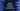# Python program to get the string between two substrings## How to get the string between two substrings in a string in python:

In this post, we will learn how to get the string between two substrings of a string in python. For that, the program will take one string, and two substrings. It will print the string between these substrings. The substrings are used as markers. One is as the start marker and the other is as the end-marker.

Let’s take a look at the below string:

``hello123|start|www.codevscolor.com|end|blahblah``

If the start substring is |start| and the end substring is |end|, it will return www.codevscolor.com, which is the string between the start and the end substring.

We can solve this problem in different ways. We can either write a regular expression or regex that will match the string and return it. Or, we can find the end index of the first substring and start index of the second substring and return the string between these two indexes. That is the result string. Also, we can use split to find the string.

### Method 1: By finding the index of the substrings:

With this method, we will find the end index of the first substring in the given string and start index of the second substring in the given string. Based on these values, we will find the substring in between them, which is the required string.

Let’s try it with the above example:

``````given_string = 'hello123|start|www.codevscolor.com|end|blahblah'
start_string = '|start|'
end_string = '|end|'

start_index = given_string.find(start_string) + len(start_string)
end_index = given_string.find(end_string)

print(given_string[start_index:end_index])``````

If you run this program, it will print the below output:

``www.codevscolor.com``

Here,

• given_string is the original string, start_string is the start substring and end_string is the end substring.
• start_index is the index of the first character after start_string. We are using find to find the first index of the start_string and adding its length to get this value.
• end_index is the index of the first character of end_index. We need the string just before this character.
• The print statement is using string slicing to find the required string. It starts a start_index and ends at end_index - 1.

### Method 2: Using a regular expression:

Regular expression or regex is the most popular way to search for a substring that matches a pattern. In our case, the pattern can be any string that starts and ends with the provided strings.

Python provides re module to work with regex. We will use that to match the pattern.

``````import re

if __name__ == '__main__':
given_string = 'hello123|start|www.codevscolor.com|end|blahblah'
start_string = '|start|'
end_string = '|end|'
pattern = '\|start\|(.*)\|end\|'

result_string = re.search(pattern, given_string).group(1)

print(result_string)``````

Here, we are using the pattern to find the string. It will print www.codevscolor.com.

### Method 3: Using split:

Splitting a string is another way to do that. We can,

• split the string at the first substring. The second part will hold the required string.
• Take the second part and again split it at the second substring. Now, the first part will hold the final result string.

So, the python program will look as like below:

``````if __name__ == '__main__':
given_string = 'hello123|start|www.codevscolor.com|end|blahblah'
start_string = '|start|'
end_string = '|end|'
result_string = given_string.split(start_string).split(end_string)

print(result_string)``````

It will print the same result.